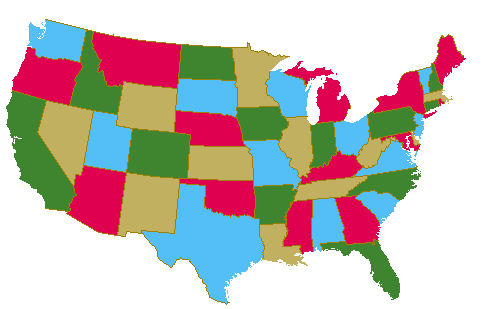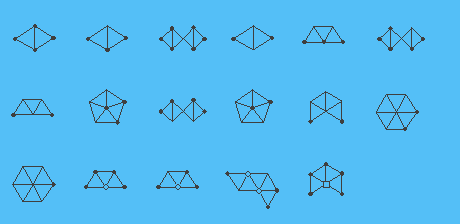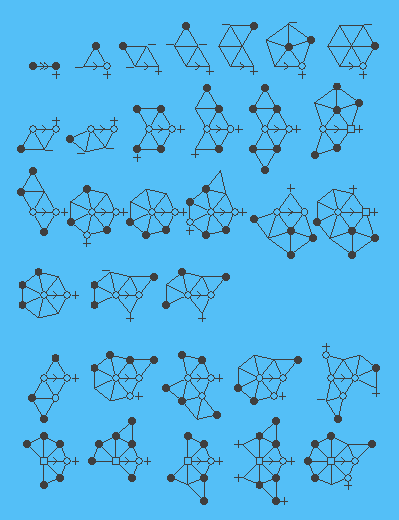# The Four Color Theorem

This page gives a brief summary of a new proof of the Four Color Theorem and a four-coloring algorithm found by Neil Robertson, Daniel P. Sanders, Paul Seymour and Robin Thomas.History.

The Four Color Problem dates back to 1852 when Francis Guthrie, while trying to color the map of counties of England noticed that four colors sufficed. He asked his brother Frederick if it was true that any map can be colored using four colors in such a way that adjacent regions (i.e. those sharing a common boundary segment, not just a point) receive different colors. Frederick Guthrie then communicated the conjecture to DeMorgan. The first printed reference is due to Cayley in 1878.

A year later the first `proof' by Kempe appeared; its incorrectness was pointed out by Heawood 11 years later. Another failed proof is due to Tait in 1880; a gap in the argument was pointed out by Petersen in 1891. Both failed proofs did have some value, though. Kempe discovered what became known as Kempe chains, and Tait found an equivalent formulation of the Four Color Theorem in terms of 3-edge-coloring.

The next major contribution came from Birkhoff whose work allowed Franklin in 1922 to prove that the four color conjecture is true for maps with at most 25 regions. It was also used by other mathematicians to make various forms of progress on the four color problem. We should specifically mention Heesch who developed the two main ingredients needed for the ultimate proof - reducibility and discharging. While the concept of reducibility was studied by other researchers as well, it appears that the idea of discharging, crucial for the unavoidability part of the proof, is due to Heesch, and that it was he who conjectured that a suitable development of this method would solve the Four Color Problem.

This was confirmed by Appel and Haken in 1976, when they published their proof of the Four Color Theorem [1,2].

Why a new proof?

There are two reasons why the Appel-Haken proof is not completely satisfactory.

• Part of the Appel-Haken proof uses a computer, and cannot be verified by hand, and
• even the part that is supposedly hand-checkable is extraordinarily complicated and tedious, and as far as we know, no one has verified it in its entirety.
We have in fact tried to verify the Appel-Haken proof, but soon gave up. Checking the computer part would not only require a lot of programming, but also inputing the descriptions of 1476 graphs, and that was not even the most controversial part of the proof.

We decided that it would be more profitable to work out our own proof. So we did and came up with a proof and an algorithm that are described below.

Outline of the proof.

The basic idea of the proof is the same as Appel and Haken's. We exhibit a set of 633 "configurations", and prove each of them is "reducible". This is a technical concept that implies that no configuration with this property can appear in a minimal counterexample to the Four Color Theorem. It can also be used in an algorithm, for if a reducible configuration appears in a planar graph G, then one can construct in constant time a smaller planar graph G' such that any four-coloring of G' can be converted to a four-coloring of G in linear time.

It has been known since 1913 that every minimal counterexample to the Four Color Theorem is an internally 6-connected triangulation. In the second part of the proof we prove that at least one of our 633 configurations appears in every internally 6-connected planar triangulation (not necessarily a minimal counterexample to the 4CT). This is called proving unavoidability, and uses the "discharging method", first suggested by Heesch. Here our method differs from that of Appel and Haken.

Main features of our proof.

We confirm a conjecture of Heesch that in proving unavoidability, a reducible configuration can be found in the second neighborhood of an "overcharged" vertex; this is how we avoid "immersion" problems that were a major source of complication for Appel and Haken. Our unavoidable set has size 633 as opposed to the 1476 member set of Appel and Haken, and our discharging method uses only 32 discharging rules, instead of the 300+ of Appel and Haken. Finally, we obtain a quadratic algorithm to four-color planar graphs (described later), an improvement over the quartic algorithm of Appel and Haken.

Configurations.

A near-triangulation is a non-null connected loopless plane graph such that every finite region is a triangle. A configuration K consists of a near-triangulation G and a map g from V(G) to the integers with the following properties:

1. for every vertex v, G\v has at most two components, and if there are two, then the degree of v is g(v)-2,
2. for every vertex v, if v is not incident with the infinite region, then g(v) equals the degree of v, and otherwise g(v) is greater than the degree of v; and in either case g(v)> 4,
3. K has ring-size at least 2, where the ring-size of K is defined to be the sum of g(v)-deg(v)-1, summed over all vertices v incident with the infinite region such that G\v is connected.

When drawing pictures of configurations we use a convention introduced by Heesch. The shapes of vertices indicate the value of g(v) as follows: A solid black circle means g(v)=5, a dot (or what appears in the picture as no symbol at all) means g(v)=6, a hollow circle means g(v)=7, a hollow square means g(v)=8, a triangle means g(v)=9, and a pentagon means g(v)=10. (We do not need vertices v with g(v)> 11, and only one vertex with g(v)=11, for which we do not use any special symbol.) In the picture below 17 of our 633 reducible configurations are displayed using the indicated convention. The whole set can be viewed by clicking here. (We refer to (3.2) of our paper  for the meaning of "thick edges" and "half-edges" in those pictures.)Any configuration isomorphic to one of the 633 configurations exhibited in  is called a good configuration. Let T be a triangulation. A configuration K=(G,g) appears in T if G is an induced subgraph of T, every finite region of G is a region of T, and g(v) equals the degree of v in T for every vertex v of G. We prove the following two statements.

THEOREM 1. If T is a minimal counterexample to the Four Color Theorem, then no good configuration appears in T.

THEOREM 2. For every internally 6-connected triangulation T, some good configuration appears in T.

From the above two theorems it follows that no minimal counterexample exists, and so the 4CT is true. The first proof needs a computer. The second can be checked by hand in a few months, or, using a computer, it can be verified in about 20 minutes.

Discharging rules.

Let T be an internally 6-connected triangulation. Initially, every vertex v is assigned a charge of 10(6-deg(v)). It follows from Euler's formula that the sum of the charges of all vertices is 120; in particular, it is positive. We now redistribute the charges according to the following rules, as follows. Whenever T has a subgraph isomorphic to one of the graphs in the figure below satisfying the degree specifications (for a vertex v of a rule with a minus sign next to v this means that the degree of the corresponding vertex of T is at most the value specified by the shape of v, and analogously for vertices with a plus sign next to them; equality is required for vertices with no sign next to them) a charge of one (two in case of the first rule) is to be sent along the edge marked with an arrow.This procedure defines a new set of charges with the same total sum. Since the total sum is positive, there is a vertex v in T whose new charge is positive. We show that a good configuration appears in the second neighborhood of v.

If the degree of v is at most 6 or at least 12, then this can be seen fairly easily by a direct argument. For the remaining cases, however, the proofs are much more complicated. Therefore, we have written the proofs in a formal language so that they can be verified by a computer. Each individual step of these proofs is human-verifiable, but the proofs themselves are not really checkable by hand, because of their length.

Pointers.

The theoretical part of our proof is described in . A 10-page survey is available on-line. The computer data and programs used to be located on an anonymous ftp server, but that server has been phased out. The same files are now available from http://people.math.gatech.edu/~thomas/OLDFTP/four/ and can be conveniently viewed. An independent set of programs was written by Gasper Fijavz under the guidance of Bojan Mohar.

The input to the algorithm will be a plane triangulation G with n vertices. (This is without loss of generality, as any planar graph can be triangulated in linear time.) The output will be a coloring of the vertices of G with four colors.

If G has at most four vertices color each vertex a different color. Otherwise if G has a vertex x of degree k < 5, then the circuit C surrounding it is a `k-ring'. Go to the k-ring analysis below. Otherwise G has minimum degree five. For every vertex we compute its charge as explained above, and find a vertex v of positive charge. It follows from our proof of Theorem 2 that either a good configuration appears in the second neighborhood of v (it which case it can be found in linear time), or a k-ring violating the definition of internal 6-connection can be found in linear time. In the latter case we go to the k-ring analysis below, in the former case we apply recursion to a certain smaller graph. A four-coloring of G can then be constructed from the four-coloring of this smaller graph in linear time.

Given a k-ring R violating the definition of internal 6-connection a procedure developed by Birkhoff can be used. We apply recursion to two carefully selected subgraphs of G. A four-coloring of G can then be constructed from the four-colorings of the two smaller graphs in linear time.

Discussion.

We should mention that both our programs use only integer arithmetic, and so we need not be concerned with round-off errors and similar dangers of floating point arithmetic. However, an argument can be made that our `proof' is not a proof in the traditional sense, because it contains steps that can never be verified by humans. In particular, we have not proved the correctness of the compiler we compiled our programs on, nor have we proved the infallibility of the hardware we ran our programs on. These have to be taken on faith, and are conceivably a source of error. However, from a practical point of view, the chance of a computer error that appears consistently in exactly the same way on all runs of our programs on all the compilers under all the operating systems that our programs run on is infinitesimally small compared to the chance of a human error during the same amount of case-checking. Apart from this hypothetical possibility of a computer consistently giving an incorrect answer, the rest of our proof can be verified in the same way as traditional mathematical proofs. We concede, however, that verifying a computer program is much more difficult than checking a mathematical proof of the same length.

Acknowledgements.

We are indebted to Thomas Fowler, Christopher Carl Heckman and Barrett Walls for their help with preparing this page. Our work was partially supported by the National Science Foundation.

References.

1. K. Appel and W. Haken, Every planar map is four colorable. Part I. Discharging, Illinois J. Math. 21 (1977), 429-490.
2. K. Appel, W. Haken and J. Koch, Every planar map is four colorable. Part II. Reducibility, Illinois J. Math. 21 (1977), 491--567.
3. K. Appel and W. Haken, Every planar map is four colorable, Contemporary Math. 98 (1989).
4. G. D. Birkhoff, The reducibility of maps, Amer. J. Math. 35 (1913), 114-128.
5. H. Heesch, Untersuchungen zum Vierfarbenproblem, Hochschulskriptum 810/a/b, Bibliographisches Institut, Mannheim 1969.
6. A. B. Kempe, On the geographical problem of the four colors, Amer. J. Math., 2 (1879), 193-200.
7. N. Robertson, D. P. Sanders, P. D. Seymour and R. Thomas, The four colour theorem, J. Combin. Theory Ser. B. 70 (1997), 2-44.
8. N. Robertson, D. P. Sanders, P. D. Seymour and R. Thomas, A new proof of the four colour theorem, Electron. Res. Announc. Amer. Math. Soc. 2 (1996), 17-25 (electronic).
9. T.L. Saaty, Thirteen colorful variations on Guthrie's four-color conjecture, Amer. Math. Monthly 79 (1972), 2-43.
10. T.L. Saaty and P. C. Kainen, The four-color problem. Assaults and conquest, Dover Publications, New York 1986.
11. P. G. Tait, Note on a theorem in geometry of position, Trans. Roy. Soc. Edinburgh 29 (1880), 657-660.
12. H. Whitney and W. T. Tutte, Kempe chains and the four colour problem'', in Studies in Graph Theory, Part II (ed. D. R. Fulkerson), Math. Assoc. of America, 1975, 378-413.

13 November 1995. Links updated 19 July 2017.

Translations: German, Romanian, Spanish courtesy of Translate Team, Greek courtesy of Dimitris Galatas.
Note to potential translators: Please do not ask me to link to your translation. I am receiving too many requests. Most of them are computer-generated and/or of low quality.